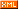# Coding Life

 博客园 :: 首页 :: 博问 :: 闪存 :: 新随笔 :: 联系 :: 订阅:: 管理 ::## 1. 声明一个指向数组的引用

int n3 = {2, 4, 6};
int (&rn3) = n3;		// A reference to an array of 3 ints


int n3 = {2, 4, 6};
typedef int Int3;
Int3& rn3 = n3;				// A reference to an array of 3 ints


## 2. 定义一个接受数组的引用作为其参数的函数

void GetInt3_Example1(int* pn3)
{
pn3 = 1;	// access the third element
}


void GetInt3_Example2(int n3)
{
n3 = 1;	// access the third element
}


int loc;	// local array of 2 integers
GetInt3_Example2(loc);	// no compile error, but access violation!


void GetInt3_Example3(Int3 n3)
{
Int3 loc	= {0};
int n3Size	= sizeof(n3);	// n3Size == 4
int locSize	= sizeof(loc);	// locSize == 12, see?
}


void GetInt3_Example4(int (&n3))


或者还是用 typedef 让它看起来舒服些？

void GetInt3_Example5(Int3& n3)


## 3. 返回一个指向数组的引用

Int3& GetInt3_Example6()
{
static int n3 = {2, 3, 1};
return n3;
}


int (&GetInt3_Example7()) 
{
static int n3 = {2, 3, 1};
return n3;
}


posted on 2011-05-04 14:52  yonken  阅读(22347)  评论(3编辑  收藏  举报